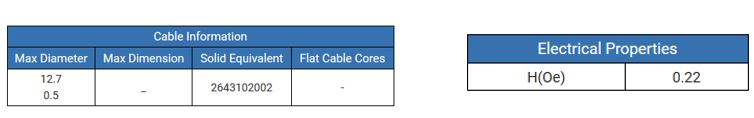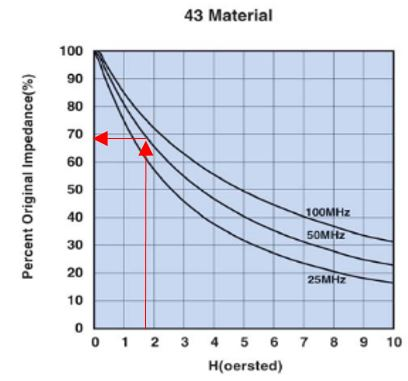Determining the Effects of DC Bias on Round Cable EMI Suppression Cores

# Determining the Effects of DC Bias on Round Cable EMI Suppression Cores

The addition of an EMI suppression core on a cable can help reduce EMI. The core is usually applied so all wires pass through the core together in what is known as common mode. In this mode electrical currents going out of the cable (through the core) also return through the cable and core. The positive and negative currents have equal and opposite magnetic flux. As a result, they negate one another and there is no static magnetic bias within the core.

However, when supply and return currents are not equal, such as in the case of a single conductor, the unidirectional current causes a biased magnetic field (H) that can degrade the operation of the EMI suppression device. It is possible to determine the level of performance under these conditions.

With little current through the conductor the EMI suppression core will demonstrate its peak impedance. As bias currents increase, the effectiveness (impedance) of the core begins to reduce. The larger the bias currents the less effective impedance the core will deliver to the circuit. To determine the effective impedance of the core under these conditions requires three steps.

1. Determine reference part number:

• For Round Cable EMI Suppression cores simply use the part number of the core.
• For Round Cable Snap-Its use the ‘Solid Equivalent’ part number. This can be found in the Fair-Rite Products catalog or on the website (www.fair-rite.com).

2. Calculate the Magnetic Field Level, H

• Determine the level of the bias current in the conductor(s) through the core.
• Locate the value of H (Oe) from catalog or part information from the internet using the reference part number.
• Multiply the current (in amperes) and the value of H (Oe). The result is the H field exposure.

3. Find the operating point on the Z vs H chart

• Find the Z vs H chart for the material from which the core is made. (The material identifier is the 3rd and 4th digits of the part number).
• Locate H on the X-Axis. Read percent of original impedance from Y-Axis.

This will show effective impedance as a result of magnetic loading within the core due to the DC bias current.

Example:
Fair-Rite Products PN 0443164151 is used on a power supply wire conducting a maximum of 8 amperes. To determine the effective impedance:

1. Reference part number (Solid Core Equivalent under the ‘Mechanical’ tab from website) is PN 2643102002.
2. From the website (Electrical tab) we find that H (Oe) value is 0.22. H is then calculated as 0.22 oersted per amp * 8 amps = 1.76 oersted.
3. From the website (Design Tools à Request Catalog à Here) we get the Z vs H chart for 43 material and determine that we can expect to still have about 68% of our rated impedance under these operating conditions.You must be signed in to use the forums